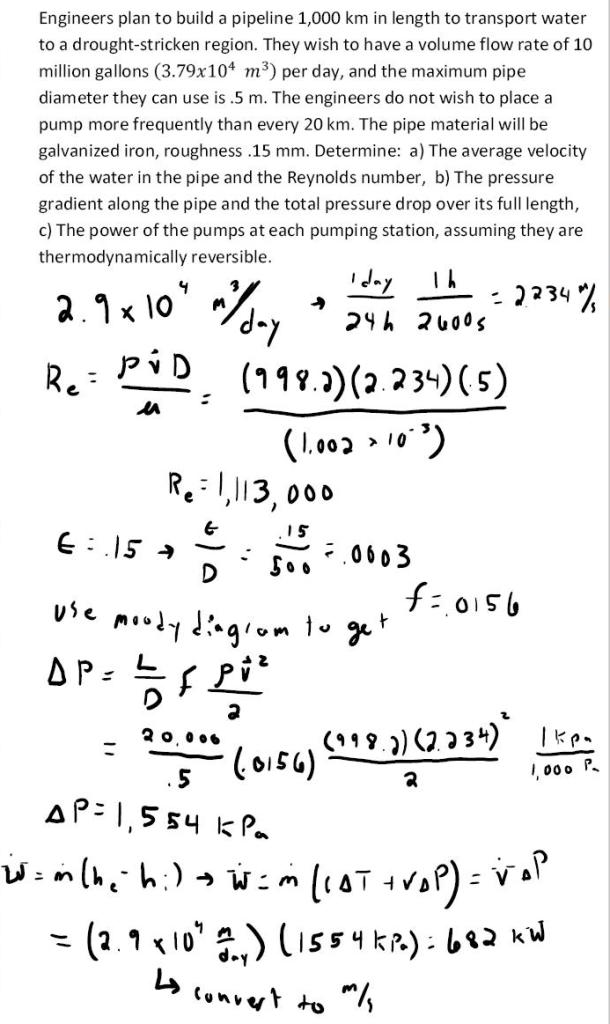fluid mechanics math problems engineering equations fluid mechanics basic mechanics school homework engineering math fluids formulas fluid mechanics problems fluid mechanics problem solutions to fluids problems full solution fluid mechanics engineering fluids problem solution fluid mechanics math problems engineering equations fluid mechanics basic mechanics school homework engineering math fluid mechanics formulas fluid problems fluid mechanics problem solutions to fluid mechanics problems full solution fluid mechanics engineering fluid mechanics problem solution
fluid mechanics math problems engineering equations fluid mechanics basic mechanics school homework engineering math fluids formulas fluid mechanics problems fluid mechanics problem solutions to fluids problems full solution fluid mechanics engineering fluids problem solution fluid mechanics math problems engineering equations fluid mechanics basic mechanics school homework engineering math fluid mechanics formulas fluid problems fluid mechanics problem solutions to fluid mechanics problems full solution fluid mechanics engineering fluid mechanics problem solution
Highalphabet Home Page fluid mechanics solutions fluids math solved Fluid Mechanics Page 1
Engineers plan to build a pipeline 1,000 km in length to transport water to a drought-stricken region. They wish to have a volume flow rate of 10 million gallons per day, and the maximum pipe diameter they can use is .5 m. The engineers do not wish to place a pump more frequently than every 20 km. The pipe material will be galvanized iron, roughness .15 mm. Determine: a) The average velocity of the water in the pipe and the Reynolds number, b) The pressure gradient along the pipe and the total pressure drop over its full length, c) The power of the pumps at each pumping station, assuming they are thermodynamically recersible.Engineers plan to build a pipeline 1,000 km in length to transport water to a drought-stricken region. They wish to have a volume flow rate of 10 million gallons per day, and the maximum pipe diameter they can use is .5 m. The engineers do not wish to place a pump more frequently than every 20 km. The pipe material will be galvanized iron, roughness .15 mm. Determine: a) The average velocity of the water in the pipe and the Reynolds number, b) The pressure gradient along the pipe and the total pressure drop over its full length, c) The power of the pumps at each pumping station, assuming they are thermodynamically recersible.